City Universe, Size

How far away is it?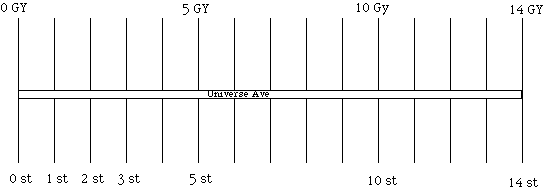Introduction

To gain an understanding of the scale of the Universe you can make a scale model the size of a city.

Material

To Do and Notice

Draw a city avenue named Universe Avenue.

Let it run for 14 blocks. Assume each block is 100 meters long.

The Universe that we see from earth reaches out 14 Billion light years to the big bang.
(At least that's the best guess in 2001 with an uncertainty of plus or minus 2 billion light years, so you might notice that those last few streets are shrouded in fog and that it's hard to count the exact number of streets.)

Each block represents a billion light years. So, a million light years is 10 cm.

Look down at your feet. Our galaxy, the Milky Way is there,100,000 light years in diameter.
In our model it is smaller than a dime, since in our model, 100,000 light years is 1 centimeter.
The galaxy has a central bulge about 10% as thick as the diameter.
In our model the central bulge is 1 mm thick, the thickness of a dime.
The disk of the galaxy is thin, about a third as thick as the bulge.
In our model it is five times thicker than a hair.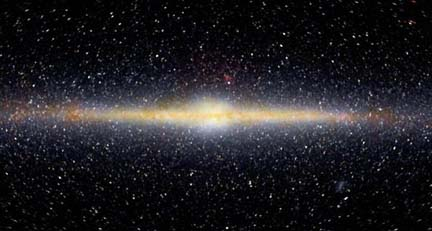An image of the Milky Way Galaxy taken from earth orbit in the infrared

The nearest large galaxy to us is the Andromeda galaxy it is 2 Million light years away.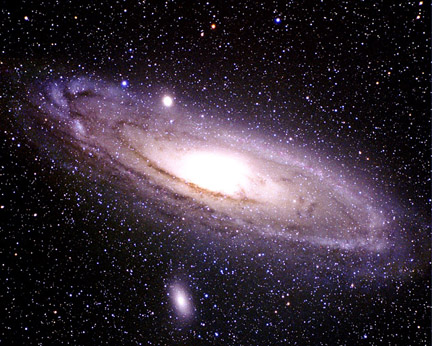Andromeda, the nearest large galaxy to the milky way.

So the Andromeda galaxy is a second dime located or 20 cm or one handspan away.

The Milky Way and the Andromeda galaxies are the largest two members of the local group of galaxies. The local group is a cluster of about 20 galaxies within 3 million light years.
20 dimes within a sphere 30 centimeters in diameter, within a sphere the size of a basketball.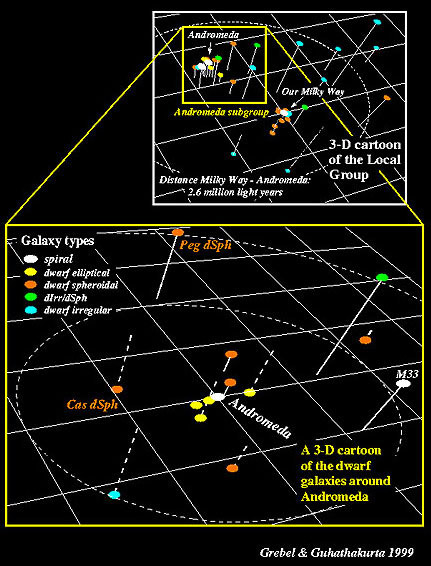An illustration of the local group from Lick Observatory.

The nearest group of galaxies is the Sculptor Group, with six bright spirals, it is 7 million light years away, or 70 cm. The galaxies in the sculptor group are all within a 3 million light year diameter, also within a basketball sized sphere (30 cm in diameter).

Further away is the Virgo cluster of galaxies, it contains over 1,000 galaxies. That's a lot of coins! It is 45 million light years away, 4.5 meters away in out model, the length of a car. It is a sphere of galaxies 80 cm in diameter the size of a giant pumpkin.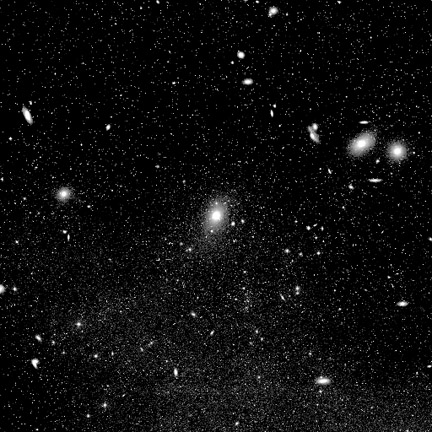The center of the Virgo Cluster.

Our local group of galaxies, the sculptor group and the Virgo cluster are members of a larger organization of galaxies called a supercluster.

Our supercluster is centered on the Virgo cluster and is roughly spherical with a diameter of about 100 million light years or 10 meters in our model. So it is a 10 meter diameter sphere centered 4.5 meters away. We are near the edge. It contains millions of galaxies. The McBean theater at the Exploratorium is one eight of a 10 meter diameter sphere, picture it full of basketball sized groups of dozens of coin-size galaxies and of giant pumpkin sized clusters of tens-of thousands of galaxies.

Superclusters are the largest spherical groupings in the universe.

If we look for structures that span 3 blocks in our model, 3 billion light years spheres, we find that one three block cube neighborhood of the universe is pretty much like every other three block cube. The universe is homogeneous, the same, from one three block group to the next it is also isotropic, meaning it looks the same in all directions.

Superclusters are organized into larger structures that form long filaments or walls, there are also huge empty regions devoid of superclusters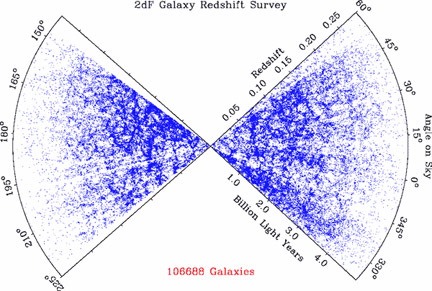The distribution of 100,000 galaxies in two wedge shaped regions of the Universe.

The most distant known objects of their kind.

The most distant Cepheid variable whose distance has been measured by the Hubble telescope is in a galaxy 108 million light years away, named NGC 4603. A tenth of a block or 10 meters distant. Twice as far as the center of the Virgo cluster.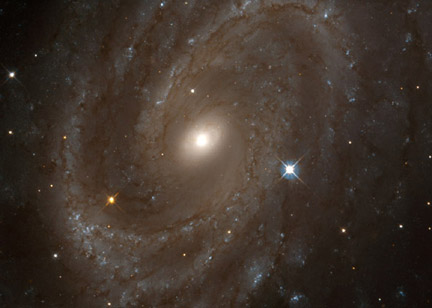Galaxy NGC 4603 which has the most distant Cepheids ever measured.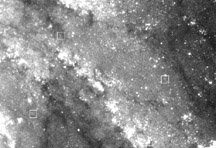The most distant Cepheids are in the boxes.

The most distant supernova of type 1a, which was measured by the Hubble telescope in 2001, has a redshift, z, of 1.7.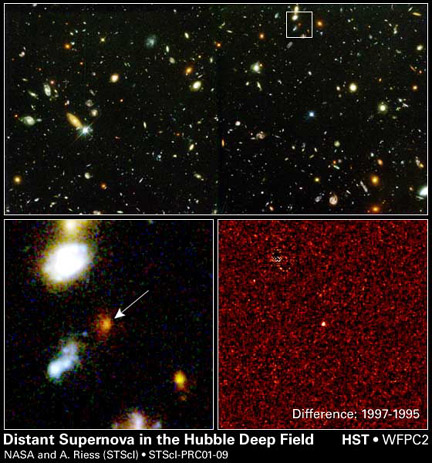The most distant supernova, 2001.

The redshift is the ratio of the change in wavelength of light as it traveled through the expanding universe to its original wavelength z = Dl/l .
The distance to this supernova can be measured because its brightness is known, its distance is 10 billion light years, or 10 blocks in our model.

The furthest galaxy clusters imaged by the Hubble telescope are estimated to be 12 billion light years, or 12 blocks, away.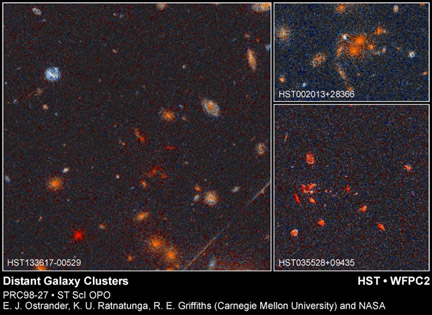Distant galaxy clusters.

The most distant object seen by the Hubble telescope is a distant galaxy imaged by the gravitational bending of light by a less distant distant cluster of galaxies. It's redshift is 5.5 and its distance is estimated to be 13.2 billion light years, just 850,000 light years from the big bang.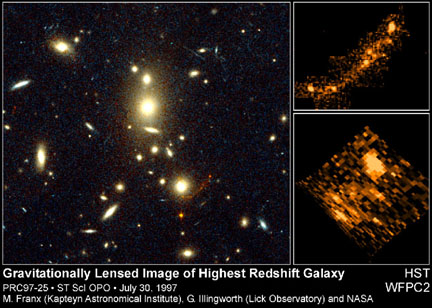The reddish arc near the middle bottom right is the gravitational lensed image of a distant galaxy.

Radio telescopes can see further, they can see the edge of the big bang, the time when it became transparent,. Just 300,000 light years shy of the big bang itself.
A few dime diameters from the edge.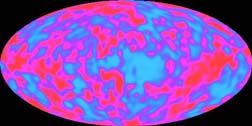An all sky image of the temperature fluctuations in the radiation from the big bang.

The City Universe

 Object Distance to its center Scale distance to its center Diameter Scale diameter Milky Way galaxy 0 0 100,000 LY 1 cm Andromeda Galaxy 2 million LY 20 cm 100,000 LY 1 cm Local Group > 20 galaxies 0 0 3 million LY 30 cm Sculptor Group 30 galaxies 7 million LY 70 cm 3 million LY 30 cm Virgo Cluster > 1,000 galaxies 45 million LY 4.5 m 8 million LY 80 cm Local Supercluster millions of galaxies 45 million LY 4.5m 100 million LY 10m Most distant cepheid 100 million LY 10 m redshift Most distant supernova 1a 11 billion LY 1100 m or 11 blocks z = 1.7 Most distant galaxy (gravity lensed) 13 billion LY 1,300 m or 13 blocks z = 5 Most distant galaxy cluster 12 billion LY 1,200 m or 12 blocks Most distant quasar 13.2 billion LY 1320 m or 13.2 blocks z = 5.5 big bang visible edge 14 billion LY 1,400 m or 14 blocks z = 1300

What's Going On?

What we measure for an object is its redshift, usually given the symbol z, where z = Dl/l, the change in wavelength, Dl, divided by the initial wavelength, l.

The redshift of a distant object is due to the expansion of the universe, the emitted photon of light has a wavelength that increases as it travels through the expanding universe. So a galaxy seen at a redshift of 0.1 means that the universe has expanded by 10% since the light from that galaxy was emitted. It also means that the universe was 1- 0.1 = 0.9 as old then as it is now. At these relatively close distances a redshift of 0.1 means a distance equal to about a tenth the distance to the big bang, which we now think is 14 billion light years, a tenth of this would be 1.4 billion light years, or 1.4 blocks away in our model.

However for larger redshifts we have to use more complex equations to calculate the fraction of the age of the universe that has passed since the light was emitted. See the Math Root.

A galaxy with a redshift of 1 means that the wavelength of light has doubled since it was emitted, and so the Universe has doubled since the time of emission.

Math Root

What astronomers actually measure for distant galaxies is their redshift, z, not their distance.

To find the expansion of the distance now, dnow between two galaxies initially at distance dthen when one is seen from the other to have redshift z the equation is dnow = dthen (1+z). We see a galaxy with a redshift 1 at a time when distances between galaxies in the universe were half what they are today. Said another way, the universe has doubled in size since the light was emitted, and so has the wavelength of the light.

We see the galaxy at a redshift z when it was some fraction, f, of the age of the universe, T.
The best current estimate for the age of the universe, T is 14 billion years +- 2 billion years.
The exact equation to calculate f when we know z depends on the model we choose for how the universe has expanded.
If gravity is slowing the universe so rapidly that it will eventually stop and recollapse it is called a closed universe.
If gravity will never stop the universe from expanding it is said to be open,
and if it is delicately balanced between these two options, so that it will expand forever while slowing toward zero growth at infinite time it is said to be flat.

Data indicates that throughout most of its expansion the universe has been approximately flat.
For a flat universe the fraction f is

f = 1/(1+z)3/2

So a galaxy of redshift z = 0.1 is seen when the universe was a fraction f = 1/(1+0.1)3/2 of its current age.
This is f = 1/(1.15) = 0.85 which, to one significant digit, is equal to 0.9 as we said above.

Light from a galaxy at a redshift of 1 left the galaxy when the universe was

f = 1/23/2 = 0.35 thirty five percent of its current age, or 5 billion years.
It is therefore 14 - 5 = 9 billion light years away.

Light with a redshift of 5 representing some of the most distant galaxies seen left when the universe was

f = 1/63/2 = .07 of its current age just under a billion years old. It is therefore 13 billion light years away.

(Note all of these distance were taken from observations up to 2001.)

Going Further

The distance to the most distant Cepheid, Supernova, galaxy, galaxy cluster, and quasar change on a monthly basis. Use the Internet to update the table above to keep up with the most recent observations.